1. /
2. CBSE
3. /
4. Class 11
5. /
6. Mathematics
7. /
8. CBSE Sample Papers Class...

# CBSE Sample Papers Class 11 Mathematics 2023

## CBSE Sample Question Paper for Class 11 Mathematics – in PDF

Students can download CBSE Sample Papers for class 11 Mathematics from the myCBSEguide app.  As we know that CBSE has released the new marking scheme and blueprint for Class 11, you can get new sample papers accordingly. We are providing Mathematics sample papers for CBSE class 11 exams. The Sample Papers are available for free download on the myCBSEguide app and website in PDF format. CBSE Sample Papers for Class 11 Mathematics with Solutions helps students to get good scores in exams.

## CBSE Sample Papers Class 11 Mathematics 2022-23

myCBSEguide provides CBSE Class 11 Sample Papers of Mathematics for the current session 2022-23 with solutions in PDF format for free download. We advise students to download new pattern model question papers and practice them regularly. Class 11 Mathematics New Sample Papers follow the blueprint of the current session only.  Students must check the latest syllabus and marking scheme. Sample papers for Class 11 Mathematics and other subjects are available for download as PDFs in our app too. myCBSEguide provides sample papers with solutions for the session 2022-23.

## Marking Scheme for the Class 11 exam

If you go through the latest model paper, you will find that CBSE has updated the marking scheme of the exam this year. Now, CBSE is asking only 2, 3 and 5 marks subjective questions. The weightage of objective-type questions if fair enough this year. So, no student can ignore them in any case. That’s why we recommend you not to follow the selective-study method. Try to cover the whole syllabus on time and revise as much as possible.

Apart from these subjective questions, CBSE will also ask MCQs, ARQs (assertion & reason)  and CBQs (Case Study) type questions.

## Class 11 – Mathematics Sample Paper – 01 (2022-23)

Maximum Marks: 80
Time Allowed: : 3 hours

General Instructions:

1. This Question paper contains – five sections A, B, C, D and E. Each section is compulsory. However, there are internal choices in some questions.
2. Section A has 18 MCQ’s and 02 Assertion-Reason based questions of 1 mark each.
3. Section B has 5 Very Short Answer (VSA)-type questions of 2 marks each.
4. Section C has 6 Short Answer (SA)-type questions of 3 marks each.
5. Section D has 4 Long Answer (LA)-type questions of 5 marks each.
6. Section E has 3 source based/case based/passage based/integrated units of assessment (4 marks each) with sub parts.

1. ### Class 11 Maths Sample Paper Section A

2. If a sin {tex}\theta{/tex} + b cos {tex}\theta{/tex} = a cosec {tex}\theta{/tex} + b sec {tex}\theta{/tex}, then the expression a2 cos6 {tex}\theta{/tex} + b2 sin6 {tex}\theta{/tex} + 2ab sin3{tex}\theta{/tex} cos3 {tex}\theta{/tex} equals:
a) 1
b) a2b2
c) 0
d) ab
3. Quartile deviation is nearly equal to
a) {tex}\frac{2}{5} \sigma {/tex}
b) {tex}\frac{2}{3} \sigma {/tex}
c) 2{tex}\sigma {/tex}
d) {tex}\frac{3}{2} \sigma {/tex}
4. Both A and B throw a dice. The chance that B throws a number not less than that thrown by A is
a) {tex}\frac{1}{2}{/tex}
b) {tex}\frac{21}{36}{/tex}
c) {tex}\frac{19}{36}{/tex}
d) {tex}\frac{15}{36}{/tex}
5. {tex}\lim \limits_{x \rightarrow \infty} \frac{|x|}{x}{/tex} is equal to
a) 0
b) 1
c) None of these
d) -1
6. In a {tex}\Delta ABC,{/tex} if A is the point (1, 2) and equations of the median through B and C are respectively x + y = 5 and x = 4, then B is
a) none of these
b) (1, 4)
c) (7, – 2)
d) (4, 1)
7. If A and B are two sets, then A {tex}\cup{/tex} (A {tex}\cap{/tex} B) is equal to
a) none of these
b) A’
c) B
d) A

To practice more questions & prepare well for exams, download myCBSEguide App. It provides complete study material for CBSE, NCERT, JEE (main), NEET-UG and NDA exams. Teachers can use Examin8 App to create similar papers with their own name and logo.

8. If |z – 2| = |z – 6| then locus of z is given by :
a) a straight line parallel to x axis
b) none of these
c) a straight line parallel to y axis
d) a circle
9. The range of the function f defined as f(x) = log5 (3x2 – 4x + 5) is
a) {tex}\left[\log _{5} \frac{11}{3}, \infty\right){/tex}
b) {tex}\left(\log _{5} \frac{11}{3}, \infty\right){/tex}
c) {tex}\left(-\infty, \log _{5} \frac{11}{3}\right]{/tex}
d) {tex}\left[-\log _{5} \frac{11}{3}, \log _{5} \frac{11}{3}\right]{/tex}
10. Given that x is an integer, find the values of x which satisfy both 2x + 3 > 7and x + 4 < 10
a) 4, 5
b) 4
c) 3, 4, 5
d) 3
11. The expression {tex}3\left[\sin ^{4}\left(\frac{3 \pi}{2}-\alpha\right)+\sin ^{4}(3 \pi+\alpha)\right]{/tex}– {tex}2\left[\sin ^{6}\left(\frac{\pi}{2}+\alpha\right)+\sin ^{6}(5 \pi-\alpha)\right]{/tex} is equal to
a) {tex}\sin 4 \alpha+\cos 6 \alpha{/tex}
b) 3
c) 1
d) 0
12. Between two junction stations A and B there are 12 intermediate stations. The number of ways in which a train can be made to stop at 4 of these stations so that no two of these halting stations are consecutive is
a) 8C4
b) 5C4
c) 9C4
d) 6C4
13. If a, b and c are distinct positive numbers, then the expression (b + c – a) (c + a – b) (a + b – c) – abc is
a) positive
b) non-negative
c) negative
d) non-positive
14. The number of terms in the expansion of {(x + a)16 + (x – a)16} is
a) 9
b) 7
c) 8
d) 17
15. Solve the system of inequalities 2x + 5 {tex}\le{/tex} 0, x – 3 {tex}\le{/tex} 0
a) {tex} x ≤ {5 \over 2}{/tex}
b) {tex} x ≥ – {5 \over 2}{/tex}
c) {tex} x ≥ {5 \over 2}{/tex}
d) {tex} x ≤ – {5 \over 2}{/tex}
16. If the sets A and B are defined as A={(x, y) : y = ex, x {tex}\in{/tex} R}; B = {(x, y) : y = x, x {tex}\in{/tex} R}, then
a) A {tex}\subseteq{/tex} B
b) B {tex}\subseteq{/tex} A
c) A {tex}\cup{/tex} B = A
d) A {tex}\cap{/tex} B = {tex}\phi{/tex}
17. Mark the Correct alternative in the following: If 2 tan{tex}\alpha{/tex} = 3 tan{tex}\beta{/tex}, then tan ({tex}\alpha{/tex}{tex}\beta{/tex}) =
a) {tex}\frac{\sin 2 \beta}{5-\cos 2 \beta}{/tex}
b) None of these
c) {tex}\frac{\cos2 \beta}{5-\cos 2 \beta}{/tex}
d) {tex}\frac{\sin 2 \beta}{5+\cos 2 \beta}{/tex}
18. Mark the correct answer for (1 + i)-1 =?
a) {tex}\left(\frac{-1}{2}+\frac{1}{2} i\right){/tex}
b) None of these
c) {tex}\left(\frac{1}{2}-\frac{1}{2} i\right){/tex}
d) (2 – i)
19. Let there be 15 letters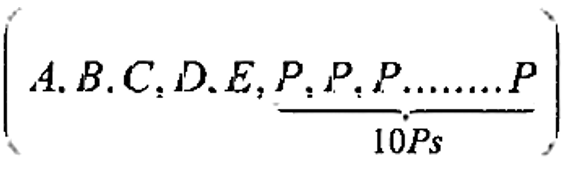. If number of arrangements of these letters in a line so that there is at least two alike letters (P) between two distinct letters is {tex}\left(\frac{1}{2}\right) k !{/tex} then the value of k is equal to:
a) 6
b) 1
c) 5
d) 7
20. If a, b {tex}\in{/tex} R and x {tex}\in{/tex} N, then
(a + b)n = {tex}{ }^n c_0 a^n+{ }^n c_1 a^{n-1} b+{ }^n c_2 a^{n-2} b^2+ { }^n c_n b^n{/tex}
Assertion (A): The no of terms in binomial expansion is (n + 1)
Reason (R): Sum of indices of a and b in each term is (n + 1)

a) Both A and R are true and R is the correct explanation of A.
b) Both A and R are true but R is not the correct explanation of A.
c) A is true but R is false.
d) A is false but R is true.
21. Assertion (A): The relation R on the set A = {1, 2, 3, 4, 5, 6} is given by R = {(1, 1), (1, 2), (1, 3), (2, 1), (2, 2), (2, 3), (3,1), (3, 2), (3, 3), (3, 4), (4, 3), (4, 4), (5, 5), (6, 6)}
Reason (R): The relation R is defined on A = {1, 2, 3, 4, 5, 6} as {(a, b) : |a2– b2 |< 9 }

a) Both A and R are true and R is the correct explanation of A.
b) Both A and R are true but R is not the correct explanation of A.
c) A is true but R is false.
d) A is false but R is true.
22. ### Class 11 Maths Sample Paper Section B

23. Let f (x) = {tex}\sqrt{x}{/tex} and g (x) = x be two functions defined in the domain R+ {tex}\cup{/tex} {0}. Find (f – g) (x).
24. Find the derivative of (x -1) (x – 2) from first principle.
25. If the abscissae and the ordinates of two points A and B be the roots of {tex}a x^{2}+b x+c=0{/tex} and a’ y2 + b’ y + c’ = 0 respectively, show that the equation of the circle described on AB as diameter is {tex}a \alpha^{\prime}\left(x^{2}+y^{2}\right)+{/tex} {tex}a^{\prime} b x+a b^{\prime} y+\left(c a^{\prime}+c^{\prime} a\right)=0{/tex}.

OR

Show that the points (x, y) given by x = {tex}\frac { 2 a t } { 1 + t ^ { 2 } }{/tex} and y = {tex}\frac { a \left( 1 – t ^ { 2 } \right) } { 1 + t ^ { 2 } }{/tex} lies on circle for all real values of t such that – 1 {tex}\leq{/tex} t {tex}\leq{/tex} 1, where a is any given real number.

26. If n(A) = 3 and n(B) = 5, find: the maximum number of elements in A {tex}\cup{/tex} B.
27. Find the equation of a line passing through the origin and making an angle of 120° with the positive direction of the x-axis.
28. ### Class 11 Maths Sample Paper Section C

29. Find the domain and range of each of the following functions given by
1. {tex}f ( x ) = \frac { 1 } { \sqrt { x – [ x ] } }{/tex}
2. {tex}f ( x ) = \frac { 1 } { \sqrt { x + [ x ] } }{/tex}
30. Find the distance of the point (-1,-5,-10) from the point of intersection of the line {tex}\vec r = \left( {2\hat i – \hat j + 2\hat k} \right) + \lambda \left( {3\hat i + 4\hat j + 2\hat k} \right){/tex}and the plane {tex}\vec r.\left( {\hat i – \hat j + \hat k} \right) = 5{/tex}

OR

Find the coordinates of the foot of perpendicular drawn from the point A (-1,8,4) to the line joining the points B (0,-1,3) and C(2,-3,-1). Hence, find the image of the point A in the line BC.

31. Find (a + b)4 – (a – b)4. Hence, evaluate {tex}{(\sqrt 3 + \sqrt 2 )^4} – {(\sqrt 3 – \sqrt 2 )^4}{/tex}

OR

Expand the given expression {tex}{\left( {\frac{x}{3} + \frac{1}{x}} \right)^5}{/tex}

32. Find the square root of i.

OR

Express {tex}\frac{(3-2 i)(2+3 i)}{(1+2 i)(2-i)}{/tex} in the form (a + ib).

33. Solve the linear inequality {tex}\frac{-3 \mathrm{x}+10}{\mathrm{x}+1}>0.{/tex}
34. How many words, with or without meaning, each of 2 vowels and 3 consonants can be formed from the letters of the word DAUGHTER.
35. ### Class 11 Maths Sample Paper Section D

36. Three coins are tossed once. Let A denote the event “three heads show”, B denote the event “two heads and one tail show”, C denote the event “three tails show” and D denote the event “a head shows on the first coin”. Which events are (i) mutually exclusive? (ii) Simple? (iii) Compound?
1. Find the derivative of {tex}\frac { \sin x + \cos x } { \sin x – \cos x }.{/tex}
2. Let {tex}f ( x ) = \left\{ \begin{array} { l l } { x ^ { 2 } – 1 , } & { 0 < x < 2 } \\ { 2 x + 3 , } & { 2 \leq x < 3 } \end{array} \right.,{/tex} find quadratic equation whose roots are {tex}\mathop {\lim }\limits_{x \to {2^ – }} f(x){/tex} and {tex}\mathop {\lim }\limits_{x \to {2^ + }} f(x).{/tex}

OR

Find the differential coefficient of sec x, using first principle.

37. If A + B + C = {tex}\pi{/tex}, prove that {tex}\sin ^{2} \frac{A}{2}{/tex} + {tex}\sin ^{2} \frac{B}{2}{/tex} + {tex}\sin ^{2} \frac{C}{2}{/tex} = 1 – {tex}2 \sin \frac{A}{2}{/tex}{tex}\sin \frac{B}{2}{/tex}{tex}\sin \frac{C}{2}{/tex}

OR

Prove that: 4 sin A sin (60o – A) sin (60o + A) = sin 3A.
Hence deduce that: sin 20o {tex}\times{/tex} sin 40o {tex}\times{/tex} sin 60o {tex}\times{/tex} sin 80o{tex}\frac { 3 } { 16 }{/tex}

38. The mean and variance of five observations are 6 and 4 respectively. If three of these are 5, 7 and 9, find the other two observations.
39. ### Class 11 Maths Sample Paper Section E

Indian track and field athlete Neeraj Chopra, who competes in the Javelin throw, won a gold medal at Tokyo Olympics. He is the first track and field athlete to win a gold medal for India at the Olympics.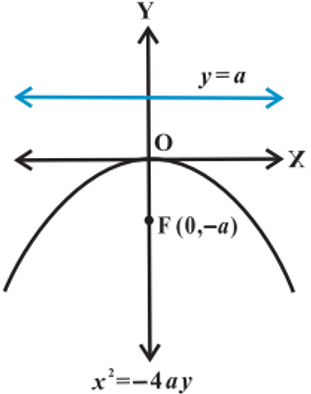1. Name the shape of path followed by a javelin. If equation of such a curve is given by x2 = -16y, then find the coordinates of foci.
2. Find the equation of directrix and length of latus rectum of parabola x2 = -16y.
3. Find the equation of parabola with Vertex (0,0), passing through (5,2) and symmetric with respect to y-axis and also find equation of directrix.
4. OR

Find the equation of the parabola with focus (2, 0) and directrix x = -2 and also length of latus rectum.

To practice more questions & prepare well for exams, download myCBSEguide App. It provides complete study material for CBSE, NCERT, JEE (main), NEET-UG and NDA exams. Teachers can use Examin8 App to create similar papers with their own name and logo.

On the roof of Monesh’s house, a water tank of capacity 9000 litres is installed. A water pump fills the tank, the pump uses water from the municipality water supply, In the beginning, the water flow of the pump remains 100 litres/hour for the first hour.
The water flow from the pump is 1.25th after each 1 hour.
Once Monesh’s mother was not at home and told him to switch off the pump when the tank is almost full.
He calculated that after how many hours should he stop the pump so water does not get overflow in the next one hour.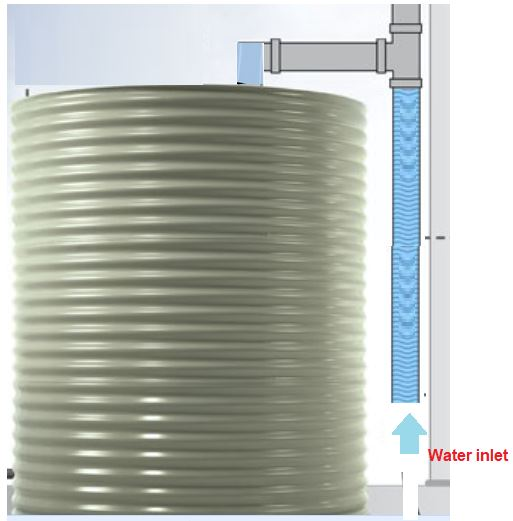1. After how many hours Monesh should stop the pump so that in the next hour the tank does not get overflow?
a) 13 hours
b) 16 hours
c) 14 hours
d) 15 hours
2. After 10 hours how much water was filled in the tank?
a) 3325.29 Liters
b) 3200 Liters
c) 3300 Liters
d) 3000 Liters
3. In 7th hour how much water was filled in the tank?
a) 375.25 Liters
b) 450 Liters
c) 400 Liters
d) 381.47 Liters
4. OR

What was the water flow in 5th hour?

a) 250 Liters/hr
b) 300 Liters/hr
c) 400 Liters/hr
d) 244.14 Liters/hr
The school organised a farwell party for 100 students and school management decided three types of drinks will be distributed in farewell party i.e., Milk (M), Coffee (C) and Tea (T).Organiser reported that 10 students had all three drinks M, C, T. 20 students had M and C; 30 students and C and T; 25 students had M and T. 12 students had M only; 5 students had C only; 8 students had T only.

1. Find the number of students who prefer Milk and Coffee but not tea?
2. Find the number of students who prefer Tea.

## Class 11 – Mathematics Sample Paper – Solution

Solution

1. ### Class 11 Maths Sample Paper Solution Section A

2. (c) 0
Explanation: a sin {tex}\theta{/tex} + b cos {tex}\theta{/tex} = {tex}\frac{a}{\sin \theta}+\frac{b}{\cos \theta}{/tex}
{tex}\Leftrightarrow{/tex} a sin2 {tex}\theta{/tex} cos {tex}\theta{/tex} + b cos2 {tex}\theta{/tex} + sin {tex}\theta{/tex}
= a cos {tex}\theta{/tex} + b sin {tex}\theta{/tex}
{tex}\Leftrightarrow{/tex} a(1 – cos2 {tex}\theta{/tex}) cos {tex}\theta{/tex} + b(1 – sin{tex}\theta{/tex}) sin {tex}\theta{/tex}
= a cos {tex}\theta{/tex} + b sin {tex}\theta{/tex}
{tex}\Leftrightarrow{/tex} -(a cos3 {tex}\theta{/tex} + b sin3 {tex}\theta{/tex}) = 0
Required expression = (a cos3 {tex}\theta{/tex} + b sin3 {tex}\theta{/tex})2
= 0
3. (b) {tex}\frac{2}{3} \sigma {/tex}
Explanation: Quartile deviation {tex}\frac{{\mathop Q\nolimits_3 – \mathop Q\nolimits_1 }}{2}{/tex} is approximately {tex}\frac{2}{3}{/tex} times the standard deviation.
4. (b) {tex}\frac{21}{36}{/tex}
Explanation: Given: B getting number not less than A means B can get number on dice greater than or equal to A.
For throwing A and B on dice, the number of elements in the sample space is 6 {tex}\times{/tex} 6 = 36 i.e. n(S) = 36
Let E be the event of “B getting number not less than A”, So it can happen in 21 ways out of 36 ways.
The ways are E = {(1, 1), (1, 2), (1, 3), (1, 4), (1, 5), (1, 6), (2, 2), (2, 3), (2, 4), (2, 5), (2, 6), (3, 3), (3, 4), (3, 5), (3, 6),(4, 4),(4, 5),(4, 6), (5, 5),(5, 6), (6, 6)} i.e. n(E) = 21
Therefore, P(E) = {tex}\frac{n(E)}{n(S)}{/tex} = {tex}\frac{21}{36}{/tex}
To practice more questions & prepare well for exams, download myCBSEguide App. It provides complete study material for CBSE, NCERT, JEE (main), NEET-UG and NDA exams. Teachers can use Examin8 App to create similar papers with their own name and logo.
5. (b) 1
Explanation: We know that,
{tex}|x|=\left\{\begin{array}{ll} x, & \text { if } x \geq 0 \\ -x, & \text { if } x<0 \end{array}\right.{/tex}
{tex}\therefore \frac{|x|}{x}=\left\{\begin{array}{ll} \frac{x}{x}, & \text { if } x \geq 0 \\ \frac{-x}{x}, & \text { if } x<0 \end{array}=\left\{\begin{array}{ll} 1, & \text { if } x \geq 0 \\ -1, & \text { if } x<0 \end{array}\right.\right.{/tex}
Now, for all {tex}x \geq 0{/tex} (however, x may large be).
{tex}\frac{|x|}{x}=1{/tex}
{tex}\therefore \lim \limits_{x \rightarrow \infty} \frac{|x|}{x}=1{/tex}
6. (c) (7, – 2)
Explanation: Let F be the midpoint on AC
Hence the coordinate of F is {tex}\frac{x_1 + 1}{2}{/tex} and {tex}\frac{5-x_1 + 2}{2}{/tex}
This point lies on x = 4
Therefore {tex}\frac{x_1 + 1}{2}{/tex} = 4
Therefore x1 = 7
Therefore y = 5 – 7 = -2
Hence the coordinates of B is (7, -2)
7. (d) A
Explanation: Let A = {1, 2, 3, 4} and B = {1, 2, 3, 4, 5, 6}
Here A {tex}\cap{/tex} B = {1, 2, 3, 4}
Now A {tex}\cup{/tex} (A {tex}\cap{/tex} B) = {1, 2, 3, 4} = A
8. (c) a straight line parallel to y axis
Explanation: |z – 2| = |z – 6|
{tex}\Rightarrow{/tex} |x – 2 + iy| = |x – 6 + iy|
{tex}\Rightarrow{/tex} {tex}\sqrt{(x-2)^{2}+y^{2}}{/tex} = {tex}\sqrt{(x-6)^{2}+y^{2}}{/tex}
{tex}\Rightarrow{/tex} x2 + 4 – 4x + y2 = x2 + 36 – 12x + y2
{tex}\Rightarrow{/tex} 8x = 32
{tex}\Rightarrow{/tex} x = 4
We have any line of the form x = a constant is a line parallel to Y-axis.
9. (a) {tex}\left[\log _{5} \frac{11}{3}, \infty\right){/tex}
Explanation: f(x) is defined, if 3x2 – 4x + 5 > 0
{tex}\Rightarrow 3\left[\left(x-\frac{2}{3}\right)^{2}+\frac{11}{9}\right]>0{/tex}, which is true for all x.
{tex}\therefore{/tex} domain of f = {tex}(-\infty, \infty){/tex}
Let y = log5 (3x2 – 4x + 5)
{tex}\Rightarrow{/tex} 5y = 3x2 – 4x + 5
{tex}\Rightarrow{/tex} 3x2 – 4x + 5 – 5y = 0
For x to be real, b2 – 4ac {tex}\ge{/tex} 0
{tex}\therefore{/tex}16 – 12(5 – 5y) {tex}\ge{/tex} 0
{tex}\Rightarrow{/tex} 12(5y) {tex}\ge{/tex} 44 {tex}\Rightarrow{/tex} (5y) {tex}\geq \frac{11}{3}{/tex}
{tex}\Rightarrow y \geq \log _{5} \frac{11}{3}{/tex}
{tex}\therefore{/tex} Range of function {tex}f=\left[\log _{e} \frac{11}{3}, \infty\right){/tex}
10. (c) 3, 4, 5
Explanation: 2x + 3 > 7
{tex}\Rightarrow{/tex} 2x + 3 – 3 > 7 – 3
{tex}\Rightarrow{/tex} 2x > 4
{tex}\Rightarrow{/tex} x > 2
Since x is an integer the solution set = {x : x > 2, x {tex}\in{/tex} Z} = {3, 4, 5, 6, 7 …..}
Now x + 4 < 10
{tex}\Rightarrow{/tex} x + 4 – 4 < 10 – 4
{tex}\Rightarrow{/tex} x < 6
Since x is an integer the solution set = {x : x > 6, x {tex}\in{/tex} Z} = {…. -1, 0, 1, 2, 3, 4, 5}
Hence the integer values which satisfy both the inequalities are {3, 4, 5, 6, 7 …..} {tex}\cap{/tex} {…. -1, 0, 1, 2, 3, 4, 5} = {3, 4, 5}
11. (c) 1
Explanation: Given expression = {tex}3\left[\sin ^{4}\left(\frac{3 \pi}{2}-\alpha\right)+\sin ^{4}(3 \pi+\alpha)\right]-2\left[\sin ^{6}\left(\frac{\pi}{2}+\alpha\right)\right.{/tex} {tex}\left.+\sin ^{6}(5 \pi-\alpha)\right]{/tex}
{tex}3\left(\cos ^{4} \alpha+\sin ^{4} \alpha\right)-2\left(\cos ^{6} \alpha+\sin ^{6} \alpha\right){/tex}
{tex}3\left(1-2 \sin ^{2} \alpha \cos ^{2} \alpha\right)-2\left(1-3 \sin ^{2} \alpha \cos ^{2} \alpha\right){/tex}
{tex}3-6 \sin ^{2} \alpha \cos ^{2} \alpha-2+6 \sin ^{2} \alpha \cos ^{2} \alpha=1{/tex}
12. (c) 9C4
Explanation: Let
x1 = number of stations before 1st halting station
x2 = between 1st and 2nd
x3 = between 2nd and 3rd
x4 = between 3rd and 4th
and x5 on the right of the 4th stations
Then, x1 {tex}\geq{/tex} 0, x5 {tex}\geq{/tex} 0, x2, x3, x4 {tex}\geq{/tex} 1
such that x1 + x2 + x3 + x4 + x5 = 8 …(i)
The total number of ways is the number of solutions of the above equation
Let y2 = x2 – 1, y3 = x3 – 1, y4 = x4 – 1. Then, equation (i) reduces to
x1 + y2 + y3 + y4 + x5 = 5, x1, y2, y3, y4 {tex}\geq{/tex} 0 …(ii)
The number of solutions to this equation is 5+5-1C5-1 = 9C4
There is bijection between two sets containing solutions of (i) and (ii)
{tex}\Rightarrow{/tex} Required number of solutions to (i) = 9C4
13. (c) negative
Explanation: Since AM > GM
{tex}\therefore{/tex} {tex}\frac{(b+c-a)+(c+a-b)}{2}{/tex} > (b + c – a) (c + a – b)1/2
{tex}\Rightarrow{/tex} c > [(b + c – a) (c + a – b)]1/2 …..(i)
Similary b > [(a + b – c) (b + c – a)]1/2 ….(ii)
and a > [(a + b – c) (c + a – b)]1/2 ….(iii)
On multiplying Eqs. (i), (ii) and (iii), we get
abc > (a + b – c) (b + c-a) (c+ a – b)
Hence,
(a + b – c)(b + c – a) (c+ a – b) – abc < 0
14. (a) 9
Explanation: Therefore,the expansion of {(x + a)16 + ( x – a)16} has {tex}\left(\frac{16}{2}+1\right){/tex} = 9 terms.
15. (d) {tex} x ≤ – {5 \over 2}{/tex}
Explanation: 2x + 5 {tex}\le{/tex} 0
{tex}\Rightarrow{/tex} 2x < -5
{tex}\Rightarrow{/tex} x {tex}\le{/tex} {tex}\frac{-5}{2}{/tex}
{tex}\Rightarrow{/tex} {tex}x \epsilon\left(-\infty, \frac{-5}{2}\right]{/tex}
Now x – 3 {tex}\le{/tex} 0
{tex}\Rightarrow{/tex} x {tex}\le{/tex} 3
{tex}\Rightarrow{/tex} {tex}x \in(-\infty, 3]{/tex}
Hence the solution set is {tex}\left( -\infty ,\frac { -5 }{ 2 } \right] \cap { \left( -\infty ,3 \right] } {/tex} = {tex}\left( -\infty ,\frac { -5 }{ 2 } \right]{/tex}
{tex}\Rightarrow{/tex}{tex} x ≤ – {5 \over 2}{/tex}
16. (d) A {tex}\cap{/tex} B = {tex}\phi{/tex}
Explanation: Since, y = ex and y = x do not meet for any x {tex}\in{/tex} R
{tex}\therefore{/tex} A {tex}\cap{/tex} B = {tex}\phi{/tex}
17. (a) {tex}\frac{\sin 2 \beta}{5-\cos 2 \beta}{/tex}
Explanation: Given 2 tan{tex}\alpha{/tex} = 3 tan{tex}\beta{/tex}
From here we get, tan{tex}\alpha{/tex} = {tex}\frac{3}{2}{/tex}tan{tex}\beta{/tex} —- (i)
since tan({tex}\alpha{/tex}{tex}\beta{/tex}) = {tex}\frac{\tan \alpha-\tan \beta}{1+\tan \alpha \tan \beta}{/tex}
tan({tex}\alpha{/tex}{tex}\beta{/tex}) = {tex}\frac{\left(\frac{3}{2} \tan \beta\right)-\tan \beta}{1+\left(\frac{3}{2} \tan \beta\right) \tan \beta}{/tex}      [ using eq (i)] tan({tex}\alpha{/tex}{tex}\beta{/tex}) = {tex}\frac{\left(\frac{3 \tan \beta-2 \tan \beta}{2}\right)}{\left(\frac{2+3 \tan ^{2} \beta}{2}\right)}{/tex}
{tex}\frac{\tan \beta}{2+3 \tan ^{2} \beta}{/tex} … [by using tan{tex}\theta{/tex} = {tex}\frac{\sin \theta}{\cos \theta}{/tex}] = {tex}\frac{\left(\frac{\sin \beta}{\cos \beta}\right)}{2+3\left(\frac{\sin \beta}{\cos \beta}\right)^{2}}{/tex}
{tex}\frac{\sin \beta \cos \beta}{2 \cos ^{2} \beta+3 \sin ^{2} \beta}{/tex}
{tex}\frac{\sin \beta \cos \beta}{2 \cos ^{2} \beta+3\left(1-\cos ^{2} \beta\right)}{/tex}
{tex}\frac{\sin \beta \cos \beta}{2 \cos ^{2} \beta+3-3 \cos ^{2} \beta}{/tex}
{tex}\frac{\sin \beta \cos \beta}{3-\cos ^{2} \beta}{/tex}
Multiplying and dividing the equation with 2
{tex}\frac{2 \sin \beta \cos \beta}{2\left(3-\cos ^{2} \beta\right)}{/tex} … [using sin2{tex}\theta{/tex} = 2 sin{tex}\theta{/tex} cos{tex}\theta{/tex}] = {tex}\frac{\sin 2 \beta}{6-2 \cos ^{2} \beta}{/tex}
In the denominator adding and subtracting 1
{tex}\frac{\sin 2 \beta}{6-2 \cos ^{2} \beta+1-1}{/tex}
{tex}\frac{\sin 2 \beta}{(6-1)-\left(2 \cos ^{2} \beta-1\right)}{/tex} … [using cos2{tex}\theta{/tex} = 2cos2{tex}\theta{/tex} – 1] tan({tex}\alpha{/tex}{tex}\beta{/tex}) = {tex}\frac{\sin 2 \beta}{5-\cos 2 \beta}{/tex}
18. (c) {tex}\left(\frac{1}{2}-\frac{1}{2} i\right){/tex}
Explanation: (1 + i)-1 = {tex}\frac{1}{(1+i)}{/tex}{tex}\frac{1}{(1+i)} \times \frac{(1-i)}{(1-i)}{/tex} = {tex}\frac{(1-i)}{\left(1^{2}-i^{2}\right)}{/tex} = {tex}\frac{(1-i)}{2}{/tex} = {tex}\left(\frac{1}{2}-\frac{1}{2} i\right){/tex}
19. (d) 7
Explanation: 7
20. (c) A is true but R is false.
Explanation: Assertion is true
{tex}\because{/tex} It is one of the observation of binomial expansion.
Reason:
Not true. AS sum of indices of a and b in each term is n.
21. (a) Both A and R are true and R is the correct explanation of A.
Explanation: Both Assertion and reason are true because R defined on the set A = {1, 2, 3, 4, 5}  by R = {(a, b) : |a2 – b2 | < 9}
For example: |1– 22| = 3 < 9 so (1 ,2) {tex}\in{/tex} R and this condition is true for the remaining element of R.
22. ### Class 11 Maths Sample Paper Solution Section B

23. Here we have the two functions, f(x) = {tex}\sqrt{x}{/tex} and g(x) = x defined in the domain {tex}R^{+} \cup\{0\}{/tex}.
Therefore, (f – g)(x) = f(x) – g(x) {tex}=\sqrt{x}-x{/tex}
24. We have, f(x) = (x – 1)(x – 2)
= x2 – 3x + 2
By first principle of derivative, we have
{tex}{f^\prime }(x) = \mathop {\lim }\limits_{h \to 0} \frac{{f(x + h) – f(x)}}{h}{/tex}
{tex} = \mathop {\lim }\limits_{h \to 0} \frac{{\left[ {{{(x + h)}^2} – 3(x + h) + 2} \right] – \left[ {{x^2} – 3x + 2} \right]}}{h}{/tex}
{tex} = \mathop {\lim }\limits_{h \to 0} \frac{{\left[ {\left( {{x^2} + {h^2} + 2xh – 3x – 3h + 2} \right] – \left[ {{x^2} – 3x + 2} \right]} \right.}}{h}{/tex}
{tex} = \mathop {\lim }\limits_{h \to 0} \frac{{2hx + {h^2} – 3h}}{h} = \mathop {\lim }\limits_{h \to 0} \frac{{h(2x + h – 3)}}{h}{/tex}
{tex}= 2 x – 3{/tex}
25. The roots of {tex}a x^{2}+b x+c=0{/tex} and a’ y2 + b’ y + c’ = 0  be x1 and x2 and y1 and y2.
{tex}x_{1}+x_{2}=-\frac{b}{a}{/tex}{tex}x_{1} x_{2}=\frac{c}{a}{/tex} ,{tex}y_{1}+y_{2}=-\frac{b^{\prime}}{a^{\prime}} \text { and } y_{1} y_{2}=\frac{c^{\prime}}{a^{\prime}}{/tex}
The equation of the circle With AB as diameter is
{tex}\left(x-x_{1}\right)\left(x-x_{2}\right)+\left(y-y_{1}\right)\left(y-y_{2}\right)=0{/tex}
{tex}x^{2}+y^{2}-x\left(x_{1}+x_{2}\right)-y\left(y_{1}+y_{2}\right)+x_{1} x_{2}+y_{1} y_{2}=0{/tex}
{tex}x^{2}+y^{2}-x\left(-\frac{b}{a}\right)-\left(-\frac{b^{\prime}}{a^{\prime}}\right) y+\frac{c}{a}+\frac{c^{\prime}}{a^{\prime}}=0{/tex}{tex}a a^{\prime}\left(x^{2}+y^{2}\right)+a^{\prime} b x+a b^{\prime} y+\left(c a^{\prime}+c^{\prime} a\right)=0{/tex}

OR

Given, x = {tex}\frac { 2 a t } { 1 + t ^ { 2 } }{/tex} and y = {tex}\frac { a \left( 1 – t ^ { 2 } \right) } { 1 + t ^ { 2 } }{/tex}
On squaring and adding, we get
x2 + y2 = {tex}\left( \frac { 2 a t } { 1 + t ^ { 2 } } \right) ^ { 2 } + \left[ \frac { a \left( 1 – t ^ { 2 } \right) } { 1 + t ^ { 2 } } \right] ^ { 2 }{/tex}
{tex}\frac { 4 a ^ { 2 } t ^ { 2 } } { \left( 1 + t ^ { 2 } \right) ^ { 2 } } + \frac { a ^ { 2 } \left( 1 – t ^ { 2 } \right) ^ { 2 } } { \left( 1 + t ^ { 2 } \right) ^ { 2 } }{/tex}
{tex}\frac { a ^ { 2 } \left( 4 t ^ { 2 } + 1 + t ^ { 4 } – 2 t ^ { 2 } \right) } { \left( 1 + t ^ { 2 } \right) ^ { 2 } }{/tex}
{tex}\frac { a ^ { 2 } \left[ t ^ { 4 } + 1 + 2 t ^ { 2 } \right] } { \left( 1 + t ^ { 2 } \right) ^ { 2 } } = \frac { a ^ { 2 } \left( t ^ { 2 } + 1 \right) ^ { 2 } } { \left( t ^ { 2 } + 1 \right) ^ { 2 } } = a ^ { 2 }{/tex}
{tex}\Rightarrow{/tex} x2 + y2 = a2
which represents a circle.
Hence proved.

26. Number of elements in set A n(A) = 3 and number of elements in set B, n(B) = 5
The number of elements in A {tex}\cup{/tex} B is n(A {tex}\cup{/tex} B).
Now for elements in A {tex}\cup{/tex} B to be maximum, there should not be any intersection between both sets that is A and B both sets must be disjoint sets as shown fig.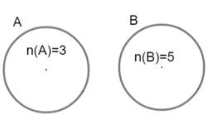Therefore, the number of elements in A {tex}\cup{/tex} B is n(A {tex}\cup{/tex} B) = n(A) + n(B)
{tex}\Rightarrow{/tex} n(A {tex}\cup{/tex} B) = 3 + 5
{tex}\Rightarrow{/tex} n(A {tex}\cup{/tex} B) = 8
Therefore,the  maximum number of elements in A {tex}\cup{/tex} B is 8.
27. As angle is given so we have to find slope first give by m = tan{tex}\theta{/tex}
m = tan120°
m = tan (180o – 60o{tex} \Rightarrow-\tan 60^{\circ}=-(\sqrt{3}){/tex}
(tan (180° – {tex}\theta{/tex}) is in II quadrant, tanx is negative)
Now we have equation of line passing through origin is given as y = mx
{tex}y=-(\sqrt{3}) x{/tex}
{tex}(\sqrt{3}) x+y=0{/tex}
Therefore, the  required equation of line is {tex}(\sqrt{3}) x+y=0{/tex}
28. ### Class 11 Maths Sample Paper Solution Section C

1. We have, f(x) {tex}= \frac { 1 } { \sqrt { x – [ x ] } }{/tex}
Domain of f
We know that, 0 {tex}\leq{/tex} x – [x] < 1 all x {tex}\in{/tex} R.
Also, x -[x] = 0 for x {tex}\in{/tex} Z.
Now, f(x) {tex}= \frac { 1 } { \sqrt { x – [ x ] } }{/tex} is defined, if
x -[x] > 0 [{tex}\because{/tex} x – [x] = 0 for x {tex}\in{/tex} Z and 0 < x – [x] < 1 for x {tex}\in{/tex} R – Z] {tex}\Rightarrow{/tex} x {tex}\in{/tex} R – Z
Hence, domain of f  = R – Z
Range of f
Put f(x) = y {tex}\Rightarrow \quad y = \frac { 1 } { \sqrt { x – [ x ] } }{/tex}
Since, {tex}x \notin Z{/tex}
{tex}\therefore{/tex} [x] < x < [x] + 1
{tex}\Rightarrow{/tex} [x] – [x] < x – [x] < 1
{tex}\Rightarrow{/tex} 0 < x – [x] < 1
{tex}\Rightarrow \quad 0 < \sqrt { x – [ x ] } < 1{/tex}
{tex}\Rightarrow \quad \frac { 1 } { \sqrt { x – [ x ] } } > 1{/tex}
{tex}\Rightarrow{/tex} y > 1
{tex}\therefore{/tex} Range of f = {tex}[ 1 , \infty ){/tex}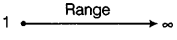2. We have, f(x) {tex}= \frac { 1 } { \sqrt { x + [ x ] } }{/tex}
Domain of f
We know that,
{tex}x + [ x ] = \left\{ \begin{array} { c } { > 0 , \text { for all } x > 0 } \\ { 0 , \text { for } x = 0 } \\ { < 0 , \text { for all } x < 0 } \end{array} \right.{/tex}
Now x + [x] > 0 {tex}\Rightarrow{/tex} x > 0
{tex}\Rightarrow \quad x \in ( 0 , \infty ){/tex}
Thus, f(x) {tex}= \frac { 1 } { \sqrt { x + [ x ] } }{/tex} is defined for all x lying in open interval (0, {tex}\infty{/tex}).
Hence, domain of f = (0, {tex}\infty{/tex})
Range of f
Let f(x) = y
{tex}\Rightarrow \quad y = \frac { 1 } { \sqrt { x + [ x ] } } , x \in ( 0 , \infty ){/tex}
{tex}\Rightarrow \quad \sqrt { x + [ x ] } = \frac { 1 } { y }{/tex}
Range of f = (0, {tex}\infty{/tex})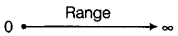29. {tex}\vec r = \left( {2\hat i – \hat j + 2\hat k} \right) + \lambda \left( {3i + 4j + 2k} \right){/tex}
{tex}\frac{{x – 2}}{3} = \frac{{y + 1}}{4} = \frac{{z – 2}}{2} = \lambda {/tex}…(i)
coordinates of any point on (i) are
{tex}3\lambda + 2,4\lambda – 1,2\lambda + 2{/tex}
and  {tex}\vec r.\left( {\hat i – \hat j + \hat k} \right) = 5{/tex}
{tex}\left( {x\hat i + y\hat j + z\hat k} \right).\left( {\hat i – \hat j + \hat k} \right) = 5{/tex}
{tex}x – y + z = 5{/tex}…(ii)
The point having coordinates {tex}3\lambda + 2,4\lambda – 1,2\lambda + 2{/tex} lies on   (ii),therefore
{tex}(3\lambda +2)-(4\lambda-1)+(2\lambda+2)=5{/tex}
{tex}\lambda = 0{/tex}
We get  (2,-1,2) as the coordinate of the point of intersection of the given line and the plane.
Given point is  {tex}\left( { – 1, – 5, – 10} \right){/tex}
req. distance {tex}= \sqrt {{{\left( {2 + 1} \right)}^2} + {{\left( { – 1 + 5} \right)}^2} + {{\left( {2 + 10} \right)}^2}} {/tex}
= 13

OR

The equation of a line joining the points {tex}B (0, -1,3)\ and\ C (2,-3,-1) {/tex} is
{tex} \vec r = (0\hat i- \hat j + 3\hat k) + \lambda [(2 – 0)\hat i + ( – 3 + 1)\hat j + ( – 1 – 3)\hat k]{/tex}
{tex} \Rightarrow \quad \vec { r } = ( – \hat { j } + 3 \hat { k } ) + \lambda ( 2 \hat { i } – 2 \hat { j } – 4 \hat { k } ){/tex}
{tex} \Rightarrow \quad \vec { r } = ( 2 \lambda ) \hat { i } + ( – 2 \lambda – 1 ) \hat { j } + ( – 4 \lambda + 3 ) \hat { k }{/tex}
Any point on line BC is to the form
{tex} \left( 2 \lambda , – 2 \lambda – 1 , – 4 \lambda + 3 \right){/tex}
Suppose foot of the perpendicular drawn from point A to the line BC be T{tex} \left( 2 \lambda ,- 2 \lambda – 1 , – 4 \lambda + 3 \right){/tex}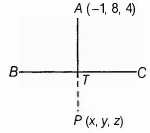Direction Ratios of line AT is {tex} ( 2 \lambda + 1 , – 2 \lambda – 1 – 8 {/tex}{tex}, – 4 \lambda – 1 ){/tex}
Direction Ratios of BC is (2-0, -3+1, -1-3) = (2, -2, -4)
Since, AT is perpendicular to BC ,
{tex} \therefore \quad 2 \times (2\lambda + 1) + ( – 2 \times ( – 2\lambda – 9) + ( – 4)( – 4\lambda – 1) = 0{/tex} [{tex}\because{/tex} {tex}a_1a_2 + b_1b_2 + c_1c_2 = 0]{/tex}
{tex} \Rightarrow \quad 4 \lambda + 2 + 4 \lambda + 18 + 16 \lambda + 4 = 0{/tex}
{tex} \lambda = – 1{/tex}
{tex}\therefore{/tex} Coordinates of foot of perpendicular is
{tex} T ( 2 \times ( – 1 ) , – 2 \times ( – 1 ) – 1 , – 4 \times ( – 1 ) + 3 ) {/tex}
=  {tex}T (-2, 1, 7){/tex}
Suppose P(x, y, z) be the image of a point A with respect to the line BC. So, point T is the mid-point of AP.
{tex}\therefore{/tex} Coordinates of T = Coordinates of mid-point of AP
{tex} \Rightarrow \quad ( – 2,1,7 ) = \left( \frac { x – 1 } { 2 } , \frac { y + 8 } { 2 } , \frac { z + 4 } { 2 } \right){/tex}
Equating the corresponding coordinates,
{tex}\Rightarrow – 2 = \frac { x – 1 } { 2 } , 1 = \frac { y + 8 } { 2 } \text { and } 7 = \frac { z + 4 } { 2 }{/tex}
{tex} \Rightarrow {/tex} {tex}x = – 3, y = – 6\ and\ z = 10{/tex}
Coordinates of the foot of perpendicular is {tex}T (-2 ,1,7){/tex} and image of the point A is {tex}P (-3, -6, 10){/tex}.

30. (a + b)4 {tex} = {[^4}{C_0}{a^4}{ + ^4}{C_1}{a^3}b{ + ^4}{C_2}{a^2}{b^2}{/tex}{tex}{ + ^4}{C_3}a{b^3}{ + ^4}{C_4}{b^4}]{/tex}
{tex}\style{font-family:Tahoma}{\style{font-size:8px}{and\;\left(a-b\right)^4{\;=\lbrack^4}C_0a^4-^4C_1a^3b+^4C_2a^2{b^2}}}{/tex}{tex}{ – ^4}{C_3}a{b^3}{ + ^4}{C_4}{b^4}]{/tex}
{tex}{\therefore\;(a+b)^4\;-\left(a-b\right)^4\;=}2\left[{}^4C_1a^3b\;+^4C_3ab^3\right]{/tex}
= 2 [4a3b +4ab3] = 8ab[a+ b2{tex}\therefore\left(\sqrt3\;+\sqrt2\right)^4\;-\;\left(\sqrt3\;-\sqrt2\right)^{4\;}{/tex}{tex}=\;8.\sqrt3.\sqrt2\left[\left(\sqrt3\right)^2+\left(\sqrt2\right)^2\right]{/tex}{tex}=\;8.\sqrt3.\sqrt2\left[3+2\right]\;=\;40.\sqrt3.\sqrt2\;=\;40\sqrt6{/tex}

OR

Using binomial theorem for the expansion of {tex}{\left( {\frac{x}{3} + \frac{1}{x}} \right)^5}{/tex} we have
{tex}{\left( {\frac{x}{3} + \frac{1}{x}} \right)^5}{/tex}{tex}{ = ^5}{C_0}{\left( {\frac{x}{3}} \right)^5}{ + ^5}{C_1}{\left( {\frac{x}{3}} \right)^4}\left( {\frac{1}{x}} \right){/tex}{tex}{ + ^5}{C_2}{\left( {\frac{x}{3}} \right)^3}{\left( {\frac{1}{x}} \right)^2}{ + ^5}{C_3}{\left( {\frac{x}{3}} \right)^2}{\left( {\frac{1}{x}} \right)^3}{/tex}
{tex}{ + ^5}{C_4}\left( {\frac{x}{3}} \right){\left( {\frac{1}{x}} \right)^4}{ + ^5}{C_5}{\left( {\frac{1}{x}} \right)^5}{/tex}
{tex} = \frac{{{x^5}}}{{243}} + 5 \cdot \frac{{{x^4}}}{{81}} \cdot \frac{1}{x} + 10 \cdot \frac{{{x^3}}}{{27}} \cdot \frac{1}{{{x^2}}}{/tex}{tex} + 10 \cdot \frac{{{x^2}}}{9} \cdot \frac{1}{{{x^3}}} + 5 \cdot \frac{x}{3} \cdot \frac{1}{{{x^4}}} + \frac{1}{{{x^5}}}{/tex}
{tex} = \frac{{{x^5}}}{{243}} + \frac{5}{{81}}{x^3} + \frac{{10}}{{27}}x + \frac{{10}}{{9x}} + \frac{5}{{3{x^3}}} + \frac{1}{{{x^5}}}{/tex}

31. Let x+ yi = {tex}\sqrt { 1 }{/tex}
Squaring both sides, we get
(x + yi)2 = i
x2 – y2 + 2xyi = i
Equating the real and imaginary parts
x2 – y2 = 0…….. (i)
{tex}2 \mathrm { xy } = 1 \Rightarrow \mathrm { xy } = \frac { 1 } { 2 }{/tex}
From the identity
{tex} (x^2+y^2)^2=(x^2-y^2)^2+4x^2y^2{/tex}
{tex} (x^2+y^2)^2{/tex}
{tex}= ( 0 ) ^ { 2 } + 4 \left( \frac { 1 } { 2 } \right) ^ { 2 }{/tex}
= 1
{tex}\therefore{/tex} x2 + y2 = 1 …….. (ii) [Neglecting (-) sign as x2 + y2 > 0] Solving (i) and (ii) we get
{tex}x ^ { 2 } = \frac { 1 } { 2 }{/tex} and {tex}\mathrm { y } ^ { 2 } = \frac { 1 } { 2 }{/tex}
{tex}\therefore { \mathrm { x } } = \pm \frac { 1 } { \sqrt { 2 } }{/tex} and {tex}\mathrm { y } = \pm \frac { 1 } { \sqrt { 2 } }{/tex}
Since the sign of xy is positive then if {tex}\mathrm { x } = \frac { 1 } { \sqrt { 2 } }, \mathrm { y } = \frac { 1 } { \sqrt { 2 } }{/tex}
If {tex}x = – \frac { 1 } { \sqrt { 2 } }, y = – \frac { 1 } { \sqrt { 2 } }{/tex}{tex}\therefore \sqrt { 1 } = \pm \left( \frac { 1 } { \sqrt { 2 } } + \frac { 1 } { \sqrt { 2 } } i \right){/tex}

OR

Given that: {tex}\frac{(3-2 i)(2+3 i)}{(1+2 i)(2-i)}{/tex}
First of all, we solve the given equation
{tex}=\frac{3(2)+3(3 i)-2 i(2)+(-2 i)(3 i)}{(1)(2)+1(-i)+2 i(2)+2 i(-i)}{/tex}
{tex}=\frac{6+9 i-4 i-6 i^{2}}{2-i+4 i-2 i^{2}}{/tex}
{tex}=\frac{6+5 i-6(-1)}{2+3 i-2(-1)}{/tex}
{tex}=\frac{6+6+5 i}{2+3 i+2}{/tex}
{tex}=\frac{12+5 i}{4+3 i}{/tex}
Now, we rationalize the above by multiplying and divide by the conjugate of 4 + 3i
{tex}=\frac{12+5 i}{4+3 i} \times \frac{4-3 i}{4-3 i}{/tex}
{tex}=\frac{(12+5 i)(4-3 i)}{(4+3 i)(4-3 i)}{/tex}
{tex}=\frac{(12+5 i)(4-3 i)}{(4)^{2}-(3 i)^{2}}{/tex}  [(a + b)(a – b) = (a2 – b2)] {tex}=\frac{12(4)+12(-3 i)+5 i(4)+5 i(-3 i)}{16-9 i^{2}}{/tex}
{tex}=\frac{48-36 i+20 i-15 i^{2}}{19-9(-1)}\left[\because i^{2}=-1\right]{/tex}
{tex}=\frac{48-16 i-15(-1)}{16+9}\left[\because i^{2}=-1\right]{/tex}
{tex}=\frac{48-16 i+15}{25}{/tex}
{tex}=\frac{63-16 i}{25}{/tex}
{tex}=\frac{63}{25}-\frac{16}{25} i{/tex}

32. We have {tex}\frac{-3 x+10}{x+1}>0{/tex}
{tex}\Rightarrow \frac{-3 x+10}{x+1} \times(x+1)^{2}>0 \cdot(x+1)^{2}{/tex}[multiplying both sides by (x + 1)2] {tex}\Rightarrow{/tex} (-3x + 10)(x + 1)>0
Therefore, Product of (-3x + 10) and (x + 1) will be positive.
Case I: if both are positive.
i.e., (-3x +10) > 0 and (x + 1)>0
{tex}\Rightarrow{/tex} 3x < 10 and x > -1
{tex}\Rightarrow \quad x<\frac{10}{3} \text { and } x>-1{/tex}
Case II: If both are negative.
i.e., (-3x + 10) < 0 and (x + 1) < 0
{tex}\Rightarrow{/tex} -3x < -10 and x < -1
{tex}\Rightarrow{/tex} 3x > 10 and x < -1
{tex}\Rightarrow \quad x>\frac{10}{3} \text { and } x<-1{/tex}
So, this is impossible. [since, system of inequalities have no common solution] Thus, the solution is {tex}\left(-1, \frac{10}{3}\right).{/tex}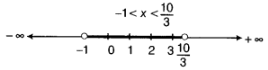33. There are 8 letters in the word DAUGHTER including 3 vowels and 5 consonants. We have to select 2 vowels out of 3 vowels and 3 consonants of 5 consonants.
{tex}\therefore {/tex} Number of ways of selection {tex}{ = ^3}{C_2}{ \times ^5}{C_3} = 3 \times 10 = 30{/tex}
Now each word contains 5 letters which can be arranged among themselves in 5! Ways.
So total number of words {tex} = 5! \times 30 = 120 \times 30 = 3600{/tex}
34. ### Class 11 Maths Sample Paper Solution Section D

35. When three coins are tossed then the sample space (S) is given by
S = {HHH, HHT, HTH, THH, TTH, THT, HTT, TTT}
A: three heads show = {HHH}
B: two heads and one tail show = {HHT, HTH, THH}
C: three tails show = {TTT}
D: a head shows on the first coin – {HHH, HHT, HTH, HTT}
(i) We know that if two events do not have common element
i.e., if intersection of two event is {tex}\style{font-size:24px}\phi{/tex} ,
then those events are called mutually exclusive events.
Here, {tex}\style{font-size:28px}{A\cap B=\phi}{/tex},   {tex}\style{font-size:28px}{B\cap C=\phi}{/tex}{tex}\style{font-size:28px}{A\cap C=\phi}{/tex} , {tex}\style{font-size:28px}{C\cap D=\phi}{/tex}
Hence, events A and B, events B and C, events A and C, events C and D
are mutually exclusive events.
(ii) A ={HHH}, Here cardinal number  = 1
{tex}\therefore{/tex}    A is a simple event.
Since, C = {TTT}, Here also cardinal number = 1
{tex}\therefore{/tex}   C is a simple event.
(iii) We see that,  B = {HHT, HTH, THH},
and D = {HHH, HHT, HTH, HTT}.
Since cardinal numbers of events B and D are respectively 3 and 4,
So, B and D are compound events.
1. Let {tex}y = \frac{{\sin x + \cos x}}{{\sin x – \cos x}}{/tex}
On differentiating both sides of y w.r.t. x, we get
{tex}\frac{{dy}}{{dx}} = \frac{{\left[ {(\sin x + \cos x)\frac{d}{{dx}}(\sin x + \cos x) – (\sin x + \operatorname{co} \left. {sx)\frac{d}{{dx}}(\sin x – \cos x)} \right]} \right.}}{{{{(\sin x – \cos x)}^2}}}{/tex}
[by quotient rule of derivative] {tex} = \frac{{[\sin x – \cos x)(\cos x – \sin x) – (\sin x + \cos x)(cos x + sin]}}{{{{(\sin x – \cos x)}^2}}}{/tex}
{tex} = \frac{{ – (\cos x – \sin x)(\cos x – \sin x) – {{(\cos x + \sin x)}^2}}}{{{{(\sin x – \cos x)}^2}}}{/tex}
{tex}= \frac { – ( \cos x – \sin x ) ^ { 2 } – ( \cos x + \sin x ) ^ { 2 } } { ( \sin x – \cos x ) ^ { 2 } }{/tex}
{tex} = \frac{{\left[ { – \left( {{{\cos }^2}x + {{\sin }^2}x – 2\cos x\sin x} \right) + ({{\cos }^2}x + {{\sin }^2}x + 2\cos x\sin x)]} \right.}}{{{{(\sin x – \cos x)}^2}}}{/tex}
{tex} = \frac{{ – [1 + 1]}}{{{{(\sin x – \cos x)}^2}}} = \frac{{ – 2}}{{{{(\sin x – \cos x)}^2}}}{/tex}
2. Given, {tex}f(x) = \left\{ {\begin{array}{*{20}{l}} {{x^2} – 1,}&{0 < x < 2} \\ {2x + 3,}&{2 \leq x < 3} \end{array}} \right.{/tex}
At x = 2,
RHL = {tex}\mathop {\lim }\limits_{x \to {2^ + }} f(x){/tex}
{tex} = \mathop {\lim }\limits_{h \to 0} f(2 + h){/tex}
{tex} = \mathop {\lim }\limits_{h \to 0}{/tex} 2(2 + h) + 3
= 2(2 + 0) + 3
= 4 + 3 = 7 = {tex}\alpha{/tex} [say] [{tex}\because{/tex} f(x) = 2x + 3] LHL = {tex}\mathop {\lim }\limits_{x \to {2^ – }} f(x) = \mathop {\lim }\limits_{h \to 0}{/tex} f(2 – h)
{tex} = \mathop {\lim }\limits_{h \to 0}{/tex} (2 – h)2 – 1 = (2 – 0)2 – 1
= 4 – 1 = 3 = {tex}\beta{/tex} [say] [{tex}\therefore{/tex} f(x) = x2 – 1] If a quadratic euation has root {tex}\alpha{/tex} and {tex}\beta,{/tex} then the equation is
x2 – (Sum of roots) x + Product of roots = 0
i.e., {tex}x ^ { 2 } – ( \alpha + \beta ) x + \alpha \beta = 0{/tex}
i.e., x2 – (7 + 3)x + 7 {tex}\times{/tex} 3 = 0
{tex}\Rightarrow{/tex} x2 – 10x + 21 = 0

OR

We have, f(x) = sec x
By using first principle of derivative,
{tex}{f^\prime }(x) = \mathop {\lim }\limits_{h \to 0} \frac{{f(x + h) – f(x)}}{h}{/tex}
{tex}\therefore {f^\prime }(x) = \mathop {\lim }\limits_{h \to 0} \frac{{\sec (x + h) – \sec x}}{h}{/tex}
{tex} = \mathop {\lim }\limits_{h \to 0} \frac{{\frac{1}{{\cos (x + h)}} – \frac{1}{{\cos x}}}}{h}{/tex}
{tex} = \mathop {\lim }\limits_{h \to 0} \frac{{\cos x – \cos (x + h)}}{{h \times \cos x \cdot \cos (x + h)}}{/tex}
{tex} = \mathop {\lim }\limits_{h \to 0} \left[ {\frac{{ – 2\sin \left( {\frac{{x + x + h}}{2}} \right) \cdot \sin \frac{{(x – x – h)}}{2}}}{{h \cdot \cos x \cdot \cos (x + h)}}} \right]{/tex}
{tex}\left[ {\because \cos C – \cos D = – 2\sin \left( {\frac{{C + D}}{2}} \right)\sin \left( {\frac{{C – D}}{2}} \right)} \right]{/tex}
{tex} = \mathop {\lim }\limits_{h \to 0} \left[ {\frac{{ – 2\sin \left( {x + \frac{h}{2}} \right) \cdot \left( { – \sin \frac{h}{2}} \right)}}{{h \cdot \cos x\cos (x + h)}}} \right]{/tex}
{tex} = \mathop {\lim }\limits_{h \to 0} \frac{{\sin \left( {x + \frac{h}{2}} \right)}}{{\cos (x + h) \cdot \cos x}} \cdot \mathop {\lim }\limits_{h \to 0} \frac{{\sin \frac{h}{2}}}{{\frac{h}{2}}}{/tex}
{tex} = \frac{{\sin x}}{{{{\cos }^2}x}} \times (1) = \frac{{\sin x}}{{\cos x}} \cdot \frac{1}{{\cos x}}{/tex}
= tanx{tex}\times{/tex}secx

36. Here it is given that, A + B + C = {tex}\pi{/tex} and we need to prove that
{tex}\sin ^{2} \frac{A}{2}{/tex} + {tex}\sin ^{2} \frac{B}{2}{/tex} + {tex}\sin ^{2} \frac{C}{2}{/tex} = 1 – {tex}2 \sin \frac{A}{2}{/tex}{tex}\sin \frac{B}{2}{/tex}{tex}\sin \frac{C}{2}{/tex}
Taking L.H.S, we have
L.H.S = {tex}\sin ^{2} \frac{A}{2}+\sin ^{2} \frac{B}{2}{/tex} + {tex}\sin ^{2} \frac{C}{2}{/tex}
{tex}\frac{1-\cos A}{2}{/tex} + {tex}\frac{1-\cos B}{2}{/tex}{tex}\frac{1-\cos C}{2}{/tex}
{tex}\frac{1-\cos A+1-\cos B+1-\cos C}{2}{/tex}
{tex}\frac{3-\cos A-\cos B-\cos C}{2}{/tex}
Using, cos A + cos A = {tex}2 \cos \left(\frac{A+B}{2}\right){/tex}{tex}\cos \left(\frac{A-B}{2}\right){/tex}
L.H.S = {tex}\frac{3-\cos A-\left\{2 \cos \left(\frac{B+C}{2}\right) \cos \left(\frac{B-C}{2}\right)\right\}}{2}{/tex}
{tex}\frac{3-\cos A-2 \cos \left(\frac{B+C}{2}\right) \cos \left(\frac{B-C}{2}\right)}{2}{/tex}
Using, since A + B + C = {tex}\pi{/tex}
= B + C = 180 – A
And, cos({tex}\pi{/tex} – A ) = -cosA
L.H.S = {tex}\frac{3-\cos A-2 \cos \left(\frac{\pi}{2}-\frac{A}{2}\right) \cos \left(\frac{B-C}{2}\right)}{2}{/tex}
{tex}\frac{3-\cos A-2 \sin \left(\frac{A}{2}\right) \cos \left(\frac{B-C}{2}\right)}{2}{/tex}
Using , cos2A = 1 – 2sin2A
L.H.S = {tex}\frac{3-1+2 \sin ^{2} \frac{A}{2}-2 \sin \frac{A}{2} \cos \frac{B-C}{2}}{2}{/tex}
{tex}\frac{2-2 \sin \frac{A}{2}\left\{\sin \frac{A}{2}-\cos \left(\frac{B-C}{2}\right)\right\}}{2}{/tex}
since A + B + C = {tex}\pi{/tex}
and Using ,
cosA – cosB = {tex}2 \sin \left(\frac{A+B}{2}\right){/tex}{tex}\sin \left(\frac{B-A}{2}\right){/tex}
L.H.S = {tex}\frac{2-2 \sin \frac{A}{2}\left\{2 \sin \left(\frac{\frac{B+C}{2}+\frac{B-C}{2}}{2}\right) \sin \left(\frac{B+C}{2}-\frac{B-C}{2}\right)\right.}{2}{/tex}
{tex}\frac{2-2 \sin \frac{A}{2}\left\{2 \sin \left(\frac{\frac{2 B}{2}}{2}\right) \sin \left(\frac{\frac{2 C}{2}}{2}\right)\right\}}{2}{/tex}
Using , since A + B + C = {tex}\pi{/tex}
L.H.S= {tex}\frac{2-2 \sin \frac{A}{2}\left\{2 \sin \left(\frac{B}{2}\right) \sin \left(\frac{c}{2}\right)\right\}}{2}{/tex}
= 1 – {tex}2 \sin \frac{A}{2} \sin \frac{B}{2}{/tex}{tex}\sin \frac{c}{2}{/tex}
= R.H.S

OR

LHS {tex}= 4 sin A\times  sin (60^o – A)\times  sin (60^o + A){/tex}
{tex}= 2 sin A [2 sin (60^o – A) sin (60^o + A)]{/tex}
= 2 sin A [cos {(60o – A) – (60o + A)} – cos {(60o – A) + (60o + A)}] [{tex}\because{/tex} 2 sin A {tex}\times{/tex} sin B = cos (A – B) – cos (A + B)] {tex}= 2 sin A [cos (- 2A) – cos 120^o]{/tex}
{tex}= 2 sin A [cos 2A – cos 120^o]{/tex} [{tex}\because{/tex} cos (- {tex}\theta{/tex}) = cos {tex}\theta{/tex}] {tex}= 2 sin A\times  cos 2A – 2 sin A \times cos 120^o{/tex}
= [sin (A + 2A) + sin (A – 2A)] – 2 sin A {tex}\left( – \frac { 1 } { 2 } \right){/tex}
[{tex}\because{/tex} {tex}2 sinA\times  cosB = sin (A + B) + sin (A – B){/tex} and cos 120o = {tex}- \frac { 1 } { 2 }{/tex}] {tex}= sin 3A + sin (- A) + sin A{/tex}
{tex}= sin 3A – sinA + sin A = sin 3A ={/tex} RHS [{tex}\because{/tex} sin (- {tex}\theta{/tex}) = – sin {tex}\theta{/tex}] {tex}\therefore{/tex} LHS = RHS
Hence proved.
Now, 4 sin A sin (60o – A) {tex}\times{/tex} sin (60o + A) = sin 3A
On putting A = 20o, we get
4 sin 20o {tex}\times{/tex} sin (60o – 20o) sin (60o + 20o) = sin 3 {tex}\times{/tex} (20o)
{tex}\Rightarrow{/tex} 4 sin 20o {tex}\times{/tex} sin 40o {tex}\times{/tex} sin 80o = sin 60o{tex}\frac { \sqrt { 3 } } { 2 }{/tex}
{tex}\Rightarrow{/tex} {tex}sin 20^o \times sin 40^o \times sin 80^o ={/tex} {tex}\frac { \sqrt { 3 } } { 8 }{/tex}
{tex}\Rightarrow{/tex} sin 20o {tex}\times{/tex} sin 40o {tex}\times{/tex} {tex}\frac { \sqrt { 3 } } { 2 }{/tex} {tex}\times{/tex} sin 80o{tex}\frac { \sqrt { 3 } } { 8 }{/tex} {tex}\times{/tex} {tex}\frac { \sqrt { 3 } } { 2 }{/tex}
[multiplying both sides by {tex}\frac { \sqrt { 3 } } { 2 }{/tex}] {tex}\therefore{/tex} {tex}sin 20^o \times sin 40^o \times sin 60^o \times sin 80^o ={/tex} {tex}\frac { 3 } { 16 }{/tex} [{tex}\because{/tex} {tex}\frac { \sqrt { 3 } } { 2 }{/tex} = sin 60o]

37. Let the other two observations be x and y
Therefore, our observations are 5, 7, 9, x and y
Mean {tex}=\frac{\text { Sum of observations }}{\text { Total number of observations }}{/tex}
{tex}6=\frac{5+7+9+x+y}{5}{/tex} {tex}\Rightarrow 6 \times 5{/tex} = 21 + x + y
{tex}\Rightarrow 30-21=x+y{/tex} or x + y = 9 ………..(i)
Now prepare the following table we have,

 xi {tex}x_{1}-\bar{x}=x_{1}-6{/tex} {tex}\left(x_{1}-\bar{x}\right)^{2}{/tex} 5 5 – 6 = -1 (-1)2 = 1 7 7 – 6 = 1 (1)2  = 1 9 9 – 6 = 3 (3)2 = x x – 6 (x – 6)2 y y – 6 (y – 6)2 {tex}\sum\left(x_{i}-\bar{x}\right)^{2}{/tex} = 11 + (x – 6)2 + (y – 6)2

So, Variance, {tex}\sigma^{2}=\frac{\sum\left(x_{1}-\bar{x}\right)^{2}}{n}{/tex}
{tex}4=\frac{11+(x-6)^{2}+(y-6)^{2}}{5}{/tex}
{tex}\Rightarrow{/tex} 20 = 11 + (x+ 36 – 12x) + (y2 + 36 – 12y)
{tex}\Rightarrow{/tex} 20 – 11 = x2 + 36 – 12x+ y2 + 36 – 12y
{tex}\Rightarrow{/tex} x2 + y2 + 72 – 12(9) – 9 = 0 from (i)] {tex}\Rightarrow{/tex} x2 + y2 + 63 – 108 = 0
{tex}\Rightarrow{/tex} x2 + y2 = 45 …………… (ii)
From eq.(i)
Now, x + y = 9
(x + y)2 = (9)2
{tex}\Rightarrow{/tex} x2 + y2 + 2xy = 81
{tex}\Rightarrow{/tex} 45 + 2xy = 81 [from (ii)] {tex}\Rightarrow{/tex} 2xy = 81 – 45 {tex}\Rightarrow{/tex} 2xy = 36
{tex}\Rightarrow{/tex} xy =18 {tex}\Rightarrow x=\frac{18}{y}{/tex} ………. (iii)
After Putting the value of x in eq. (i) we get
{tex}\Rightarrow \frac{18}{y}+y=9 {/tex}{tex}\Rightarrow \frac{18+y^{2}}{y}=9{/tex} {tex}\Rightarrow{/tex} y2 + 18 = 9y
{tex}\Rightarrow{/tex} y2 – 9y + 18 = 0 {tex}\Rightarrow{/tex} y2 – 6y – 3y + 18 = 0
{tex}\Rightarrow{/tex} y(y – 6) – 3(y – 6) = 0 {tex}\Rightarrow{/tex} (y – 3) (y – 6) = 0
{tex}\Rightarrow{/tex} y – 3 = 0 and y – 6 = 0 {tex}\Rightarrow{/tex} y = 3 and y = 6
For y = 3
{tex}x=\frac{18}{y}=\frac{18}{3}=6{/tex}
Hence, x = 6, y = 3 are the remaining  two observation
For y = 6
{tex}x=\frac{18}{y}=\frac{18}{6}=6{/tex}
Hence, x = 3, y = 6 are the remaining two observation
Therefore, remaining two observations are 3 and 6
To practice more questions & prepare well for exams, download myCBSEguide App. It provides complete study material for CBSE, NCERT, JEE (main), NEET-UG and NDA exams. Teachers can use Examin8 App to create similar papers with their own name and logo.

38. ### Class 11 Maths Sample Paper Solution Section E

1. The path traced by Javelin is parabola. A parabola is the set of all points in a plane that are equidistant from a fixed line and a fixed point (not on the line) in the plane.
compare x2 = -16y with x2 = -4ay
{tex}\Rightarrow{/tex} – 4a = -16
{tex}\Rightarrow{/tex} a = 4
coordinates of focus for parabola x2 = -4ay is (0, -a)
{tex}\Rightarrow{/tex} coordinates of focus for given parabola is (0, -4)
2. compare x2 = -16y with x2 = -4ay
{tex}\Rightarrow{/tex} -4a = -16
{tex}\Rightarrow{/tex} a = 4
Equation of directrix for parabola x2 = -4ay is y = a
{tex}\Rightarrow{/tex} Equation of directrix for parabola x2 = -16y is y = 4
Length of latus rectum is 4a = 4 × 4 = 16
3. ​​​Equation of parabola with axis along y – axis
x2 = 4ay
which passes through (5, 2)
{tex}\Rightarrow{/tex} 25 = 4a {tex}\times{/tex} 2
{tex}\Rightarrow{/tex} 4a = {tex}\frac{25}{2}{/tex}
hence required equation of parabola is
{tex}x^2=\frac{25}{2} y{/tex}
{tex}\Rightarrow{/tex} 2x2 = 25y
Equation of directrix is y= -a
Hence required equation of directrix is 8y + 25 = 0.
4. OR

Since the focus (2,0) lies on the x-axis, the x-axis itself is the axis of the parabola.
Hence the equation of the parabola is of the form either y2 = 4ax or y2 = -4ax.
Since the directrix is x = -2 and the focus is (2,0), the parabola is to be of the form y2 = 4ax with a = 2.
Hence the required equation is y2 = 4(2)x = 8x
length of latus rectum = 4a = 8

1. (c) 14 hours
Explanation: 14 hours
2. (a) 3325.29 Liters
Explanation: 3325.29 Liters
3. (d) 381.47 Liters
Explanation: 381.47 Liters
4. OR

(d) 244.14 Liters/hr
Explanation: 244.14 Liters/hr

1.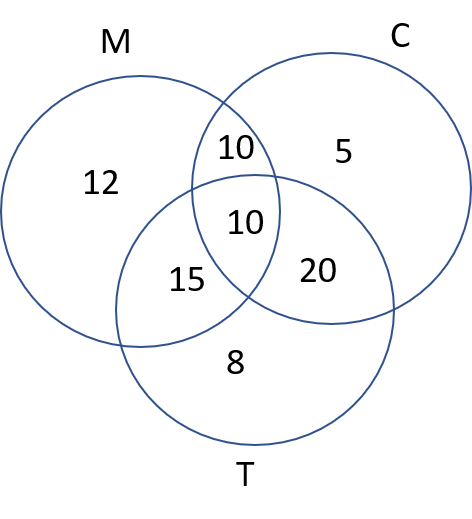only n(M {tex}\cap{/tex} C) not tea = n(M {tex}\cap{/tex} C) – n(M {tex}\cap{/tex} C {tex}\cap{/tex} T)
{tex}\Rightarrow{/tex} only n(M {tex}\cap{/tex} C) not tea = 20 – 10 = 10​​​​​
The number of students who prefer Milk and Coffee but not tea = 10
2.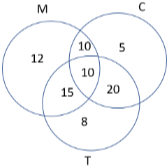n(T) = only n(T) + n(M {tex}\cap{/tex} T) + n(T {tex}\cap{/tex} C) – n(M {tex}\cap{/tex} C {tex}\cap{/tex} T)
{tex}\Rightarrow{/tex} n(T) = 8 + 25 + 30 – 10 = 53​​​​​​
The number of students who prefer Tea = 53

## CBSE Sample Papers for Class 11 2023

To download sample paper for class 11 Physics, Chemistry, Biology, History, Political Science, Economics, Geography, Computer Science, Home Science, Accountancy, Business Studies and Home Science; do check myCBSEguide app or website. myCBSEguide provides sample papers with solution, test papers for chapter-wise practice, NCERT solutions, NCERT Exemplar solutions, quick revision notes for ready reference, CBSE guess papers and CBSE important question papers. Sample Paper all are made available through the best app for CBSE students and myCBSEguide website.### Test Generator

Create question paper PDF and online tests with your own name & logo in minutes.### myCBSEguide

Question Bank, Mock Tests, Exam Papers, NCERT Solutions, Sample Papers, Notes